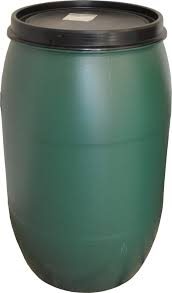# Full of water

From a tank full of water, a man removed 2/7 of it. If it now contains 450 liters, how many liters does it hold when full?

V =  630 l

### Step-by-step explanation:Did you find an error or inaccuracy? Feel free to write us. Thank you!

Math student
Where did the 5 in the 5V come from?

Dr Math
multiply both sides by 7 to avoid fractions. Then 7V-2V = 5V

Tips for related online calculators
Need help calculating sum, simplifying, or multiplying fractions? Try our fraction calculator.
Do you have a linear equation or system of equations and looking for its solution? Or do you have a quadratic equation?